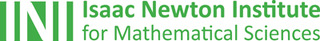## The Isaac Newton Institute: Creating eureka momentsOne of the most exciting places in the mathematical world is the Isaac Newton Institute for Mathematical Sciences (INI), an international research centre and our neighbour here on the University of Cambridge's maths campus.
The INI attracts leading mathematical scientists from all over the world, and is open to all. We are proud to be collaborating with the INI to bring the cutting edge mathematics that is being done there to the general public. The following content is part of this collaboration.

Maths in a minute: QALYs

What's a quality adjusted life year, or QALY?

Pandemics and psychology

Can you capture people's behaviour in epidemiological models?

Maths in a Minute: Turbulence and the Reynolds number

How does a smooth flow suddenly become turbulent? The Reynolds number helps understand the transition.

In this year's advent calendar we celebrate our collaboration with the Isaac Newton Institute for Mathematical Sciences.

Maths in a Minute: Fluid dynamics and the Euler equations

How does water, or indeed any fluid, move? The Euler equations let us look beneath the surface and mark the beginning of modern fluid dynamics.

Maths in a minute: Asymptotic expansions

Approximating a function with a divergent series.

The power of small things: Resurgent asymptotics

If you ignore exponentially small terms they can come back to bite you.